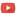KE8QZC | SFW | TSWIf you can answer any of these questions, let's write a paper!

## Special functions

1. The hypergeometric Legendre difference equation is $t(t-1)\Delta^2 y(t-2) + 2t \Delta^2 y(t-1) + 2t \Delta y(t-1) + 2\Delta y(t) - n(n+1)y(t)=0,$ which is equivalent to $(2t+6)\Delta^3 y(t) + (t^2+7t+14-n(n+1))\Delta^2 y(t) + (10t+30-2n(n+1))\Delta y(t) + (8t+24-n(n+1))y(t)=0.$ We know a polynomial solution when $n \in \{1,2,\ldots\}$, as well as numerous properties of these functions. Similar situations exist for the discrete Chebyshev and discrete polylogarithm functions.
Question: What can be determined about the other linearly independent solution(s) of the Chebyshev, hypergeometric Legendre, and polylogarithm difference equations? What about solutions of non-integer order? What about their properties?
2. In the theory of matrix hypergeometric functions, the polynomial sequence $p_1(\lambda) = \dfrac{1}{k_0}, \quad p_{j+1}(\lambda) = p_j(\lambda)\left( 1 + \dfrac{\lambda}{k_0+k} \right)$
3. arises. With the assumption that $\lambda > 0$, it is easy to see that $p_k(\lambda)$ is bounded below by a harmonic series.
Question: Can you find a lower bound for $p_k(\lambda)$ when $\lambda < 0$?
4. Two natural matrix hypergeometric series are studied in the literature: the ones emanating from Jodar and Cortes which begin with the hypergeometric power series and the ones emanating from Tirao which begin with the matrix analogue of the hypergeometric differential equations. If the parameter matrices all commute, then these two functions are equivalent, but they are not equivalent otherwise. A series for the Tirao-type is known and some differential equations for the Jodar and Cortes type are known, but not a general formula.
Question: Can you find a general differential equation (likely in a factored form) for the Jodar and Cortes-type matrix hypergeometric functions?
5. Question: How can discrete special functions be further generalized? e.g. to time scales or broader classes of functions
Relevant references
• Tom Cuchta and Dallas Freeman. Discrete polylogarithm functions. Tatra Mt. Math. Publ., 2022. In press.
• Tom Cuchta, David Grow, and Nick Wintz. Discrete matrix hypergeometric functions. J. Math. Anal. Appl., 518(2):126716, 2023.
• Tom Cuchta, David Grow, and Nick Wintz. Divergence criteria for matrix generalized hypergeometric series. Proc. Amer. Math. Soc., 150(3):1235–1240, 2022.
• Tom Cuchta, Rebecca Luketic. Discrete Hypergeometric Legendre Polynomials. Mathematics 9(20), 2546, 2021.
• Tom Cuchta, Michael Pavelites, Randi Tinney. The Chebyshev Difference Equation. Mathematics 8(1), 74, 2020.
• Martin Bohner, Tom Cuchta. The generalized hypergeometric difference equation. Demonstratio Mathematica, 51(1), pp. 62–75 (2018).
• Martin Bohner, Tom Cuchta. The Bessel difference equation. Proc. Amer. Math. Soc. 145 (2017), no. 4, 1567–1580.

## Probability theory on time scales

1. It is known that the time scales Taylor monomials $h_k(t,s)$ are of exponential order $\alpha$. This fact was used to estimate bounds of the expected value $\mathbb{E}[h_n(G,s)]$, where $G$ is a dynamic Gompertz random variable. It would be nice to compute a similar estimate for the traditional moments of $G$.
Question: Is the function $t \mapsto (t-s)^n$ of exponential order $\alpha$ on all time scales for every $n \in \{1,2,3,\ldots\}$? If not, under what restricted class of time scales are they of exponential order $\alpha$?
2. It is known that as $h \rightarrow 0$, the Gompertz distribution on $h\mathbb{N}_0$ converges pointwise to the classical Gompertz distribution on $[0,\infty)$.
Question: Does the same hold true for the Gompertz distributions on $h\mathbb{Z}$ and $\mathbb{R}$? Can the type of convergence be improved (not pointwise, but...?)
3. Question: Do the various theorems that characterize the normal distribution generalize to time scales? If they do, then do they induce the same or different distributions?
Relevant references
• Tom Cuchta and Robert J. Niichel. Memoryless properties on time scales. Math. Slovaca, 2022. In press.
• Umit Aksoy, Tom Cuchta, Svetlin Georgiev, and Yeliz Okur. A normal distribution on time scales with application. Filomat. In press.
• Tom Cuchta, Robert Jon Niichel, Sabrina Streipert. A Gompertz distribution for time scales. Turk J. Math., 45(1):185-200, 2021.

## General time scales calculus

1. Can you express $\mathscr{L}_{\mathbb{T}}\{f^{\sigma}\}(z;s)$ in a general way?
2. What can be determined about the limit as $t \rightarrow \infty$ of the solution of the dynamic Gompertz model? Currently we can bound the limit, but can it be expressed in closed form?
3. What theorems of the equation $\left(y^{\Delta}p\right)^{\Delta}+qy^{\sigma}=f$ extend to the case of conformable calculus on time scales?
4. What are the Riemann-Liouville and Caputo fractional derivatives on an arbitrary time scale?
5. How can the definition of a periodic function on an isolated time scale extend to all time scales?
6. Is there an analogue of the Bohr-Mollerup theorem for the time scales gamma function?
Relevant references
• Tom Cuchta and Nick Wintz. Periodic functions related to the Gompertz difference equation. Math. Biosci. Eng., 19(9):8774–8785, 2022.
• Martin Bohner, Tom Cuchta, and Sabrina Streipert. Delay dynamic equations on isolated time scales and the relevance of one-periodic coefficients. Math. Meth. Appl. Sci., 45(10):5821–5838, 2022.
• Tom Cuchta, Svetlin Georgiev. Analysis of the bilateral Laplace transform on time scales with applications. Int. J. Dyn. Syst. Differ. Equ. Vol. 11, Nos. 3/4, pp.255–274, 2021.
• F. Ayça Çetinkaya, Tom Cuchta. Sturm-Liouville and Riccati Conformable Dynamic Equations. Adv. Dyn. Syst. Appl., 15(1):1–13, 2020.
• Tom Cuchta, David Grow, Nick Wintz. A dynamic matrix exponential via a matrix cylinder transformation. J. Math. Anal. Appl., 479(1):733–751, 2019.